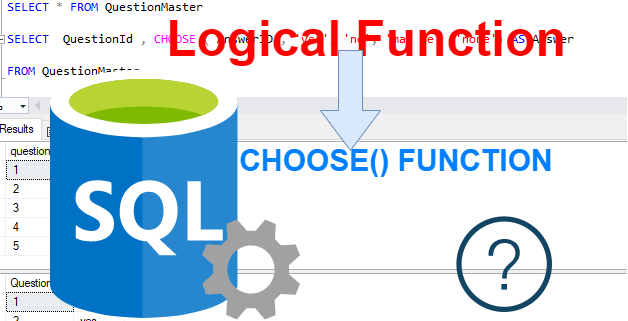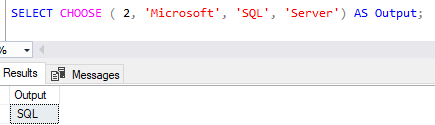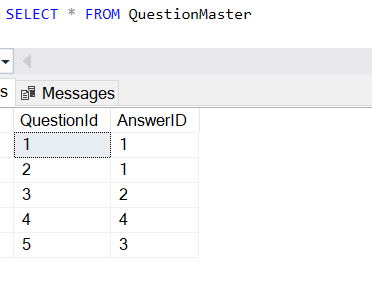Home » SQL Server CHOOSE Function

# SQL Server CHOOSE Function### SQL Server CHOOSE() Functions is the logical function that returns the item at the specified index from a list of values in SQL Server.

#### Syntax

```CHOOSE (index, val_1, val_2 [, val_n ] )
```

index
It is an integer expression that represents a 1-based index into the list of the items following it.

If index is 1, then CHOOSE() function returns val_1. If index is 2, the CHOOSE() function returns val_2, and so on.

If the provided index value has a numeric data type other than int, then the value is implicitly converted to an integer. If the index value exceeds the bounds of the array of values, then CHOOSE returns null.

val_1 … val_n
List of comma separated values of any data type.

Lets Look at an example of CHOOSE Function in SQL Server.

#### CHOOSE () Function

The following statement uses CHOOSE function and returns the second item from the list of values that is given.

```
SELECT CHOOSE ( 2, 'Microsoft', 'SQL', 'Server') AS Output;```#### Using CHOOSE() Function With table column

First we create a table then insert some records in table.

```CREATE TABLE QuestionMaster
(QuestionId INT NOT NULL, AnswerID INT)

INSERT INTO QuestionMaster
VALUES
( 1, 1),(2, 1),(3, 2),(4, 4),(5, 3)```Now we have a table QuestionMasterFollowing statement uses the CHOOSE() function to return the Answer based on the value of AnswerId column from QuestionMaster table .

```SELECT * FROM QuestionMaster

SELECT QuestionId , CHOOSE( AnswerID , 'yes', 'no', 'may be' ,'none') AS Answer

FROM QuestionMaster```This site uses Akismet to reduce spam. Learn how your comment data is processed.